PSAT Math : Whole and Part

Example Questions

2 Next →

Example Question #5 : How To Find The Part From The Whole

Mikey has one full box of cereal.  On Saturday, he eats 1/3 of the the box.  On Sunday he eats 2/3 of what is left.  How much of the box is still left?

2/3

2/9

4/9

none

5/9

2/9

Explanation:

If Mikey eats 1/3 of the box, he is left with 1 – 1/3 = 2/3.

When he eats 2/3 of what remains, he is eating 2/3 of 2/3, or 4/9 of the box.

2/3 – 4/9 = 2/9

Example Question #6 : How To Find The Part From The Whole

What is the remainder when 27 is divided by 6?

4.5

4

8

0.5

3

3

Explanation:

Long division is the fastest way: 6 goes into 27 four times. 6 times four is 24. 27 – 24 = 3.

Example Question #1 : How To Find The Part From The Whole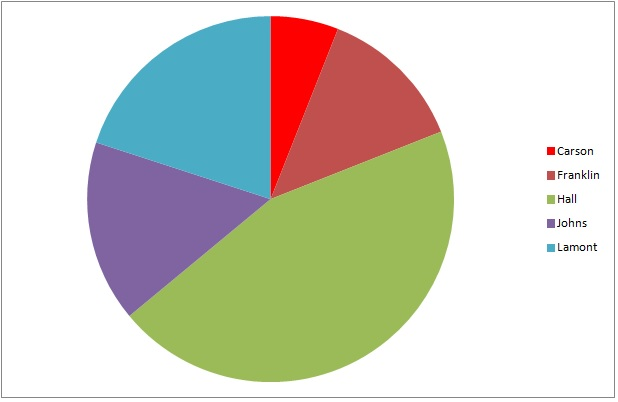The above graph represents the results of a general election for mayor of Kingston.

If 6,239 people voted in the election, which is closest to the number of people who voted for Johns?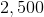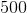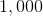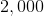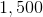Explanation:

The dark purple wedge, which represents Johns, is about one-sixth of the circle, so roughly one-sixth of the voters chose him. This is about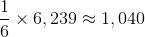so 1,000 is the best choice among the ones given.

Example Question #2 : How To Find The Part From The Whole

Kim weighs two-thirds as much as Jim. Jim weighs 50 pounds more than Tim. Together, Kim, Jim and Tim weight 430 pounds. How much do Jim and Kim weigh together?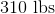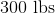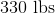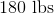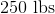Explanation:

Create an equation to represent the combined weights, in pounds, of Kim, Jim and Tim.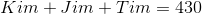Now, taking the other information we are given, put the weights of Kim and Tim in terms of Jim:

Kim weighs two-thirds of Jim: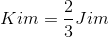Jim weighs 50 pounds more than Tim is the same as saying that Tim weighs 50 pounds less than Jim: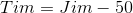Replace the variables of Kim and Jim in the equation with their substitutions: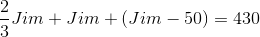Solve for Jim's weight. To combine the fractions, put all variables in terms of thirds and combine like variables: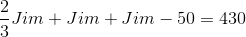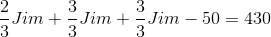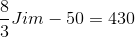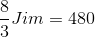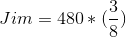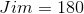Jim weighs 180 pounds. Kim weighs two-thirds of 180: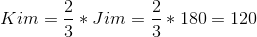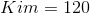The combined weight of Jim and Kim is equal to: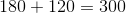Example Question #3 : How To Find The Part From The Whole

There are 60 ties on a rack in the department store. One-third are blue, two-fifths are green, and the remainder are red. How many red ties are on the rack in the department store?

11

14

12

16

18

16

Explanation:

Begin by finding how many blue and green ties there are in the department store. If one-third of 60 ties are blue, then there are 20 blue ties: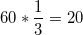Addtionally, if two-fifths of the ties are green, then there are 24 green ties: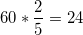To find the number of red ties, subtract the green and blue ties from the total number: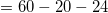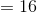There are 16 red ties in the department store.

Example Question #7 : How To Find The Part From The Whole

A pitcher is currentlyof the way full of water.  Ifounces are poured out, then the pitcher would beof the way full.  How many ounces of water are in the pitcher before theounces are poured out?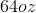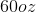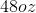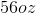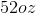Explanation:

Let p represent the total capacity of the pitcher.  The current volume in the pitcher is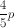.  If this volume is depleted byounces, it becomes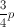.  In other words: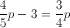Solve this equation for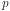and get: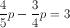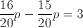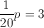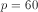Remember,represents the total capacity of the pitcher, or 60 ounces.  The question asks how many ounces are in the pitcher at the beginning of the problem, so evaluate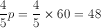.

2 Next →